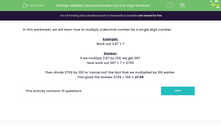# Multiply Decimal Numbers by One-Digit Numbers

In this worksheet, students practise multiplying a decimal number by a single digit number by 'getting rid of the decimal' first.Key stage:  KS 2

Curriculum topic:   Number: Fractions, Decimals and Percentages

Curriculum subtopic:   Multiply with Two Decimal Places

Difficulty level:#### Worksheet Overview

In this worksheet, we will learn how to multiply a decimal number by a single digit number.

Example:

Work out 3.87 × 7

If we multiply 3.87 by 100, we get 387.

Now work out 387 × 7 = 2709

Then divide 2709 by 100 to 'cancel out' the fact that we multiplied by 100 earlier.

This gives the answer 2709 ÷ 100 = 27.09

### What is EdPlace?

We're your National Curriculum aligned online education content provider helping each child succeed in English, maths and science from year 1 to GCSE. With an EdPlace account you’ll be able to track and measure progress, helping each child achieve their best. We build confidence and attainment by personalising each child’s learning at a level that suits them.

Get started